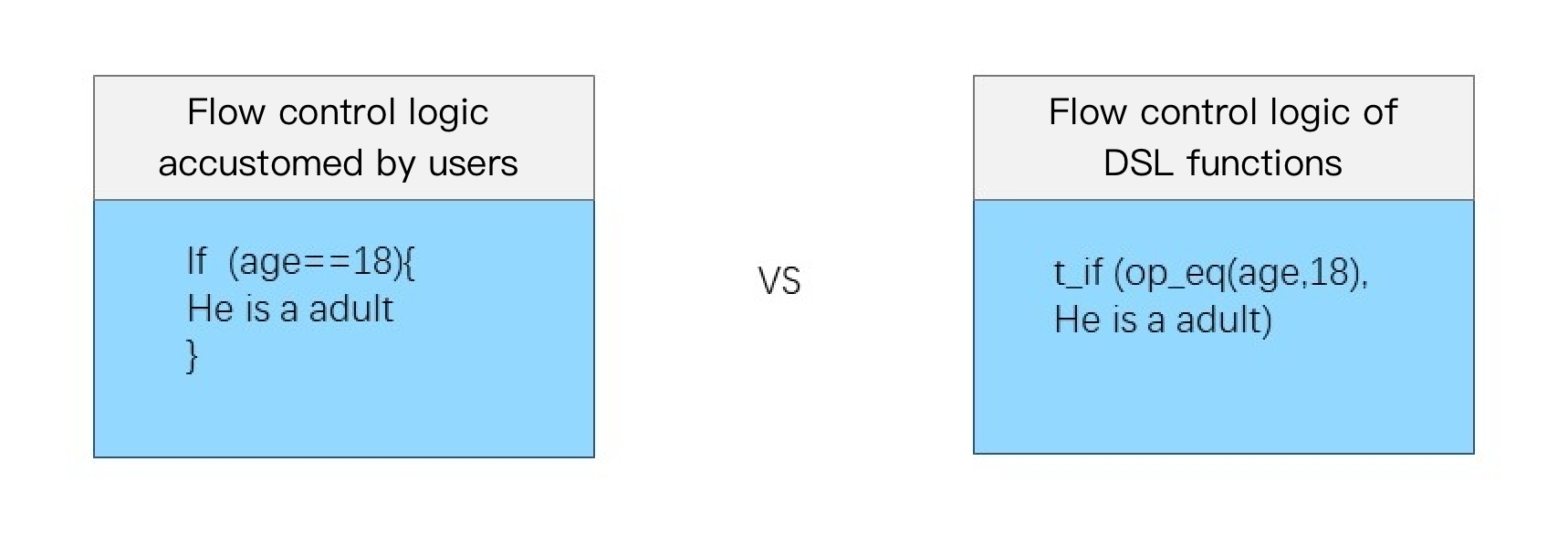# tencent cloud

### Flow Control

Last updated: 2022-04-19 16:22:15

## Overview

The writing method of the flow control logic in commonly used programming languages is different from that in DSL functions. See the figure below.## Function compose

#### Function definition

This function is used to combine multiple operation functions. Providing combination capabilities similar to those of branch code blocks, this function can combine multiple operation functions and execute them in sequence. It can be used in combination with branches and output functions.

#### Syntax description

``````compose(Function 1,Function 2, ...)
``````

#### Parameter description

Parameter Description Parameter Type Required Default Value Value Range
Variable parameter, function The parameter must be a function whose return value type is LOG. string At least one function parameter - -

#### Example

• Example 1. Call functions in sequence, executing the `enrich` function first and then the `fields_set` function
Raw log:
``````{"status": "500"}
``````

Processing rule:

``````// 1. `enrich` function: use the data in `dict` to enrich the raw log, where `status` is `500`, and generate a field (field `message` with value `Failed`) after the enrichment.
//2. `fields_Set` function: add a field `new` and assign value `1` to it.
compose(enrich_dict("{\"200\":\"SUCCESS\",\"500\":\"FAILED\"}", "status", output="message"), fields_set("new", 1))
``````

Processing result:

``````// The final log contains 3 fields:
{"new":"1","message":"FAILED","status":"500"}
``````
• Example 2
Raw log:
``````{"status": "500"}
``````

Processing rule:

``````compose(fields_set("new", 1))
``````

Processing result:

``````{"new":"1","status":"500"}
``````
• Example 3
Raw log:
``````{"condition1": 0,"condition2": 1, "status": "500"}
``````

Processing rule:

``````t_if_else(v("condition2"), compose(fields_set("new", 1),log_output("target")), log_output("target2"))
``````

Processing result, `target` output:

``````{"new":"1","condition1":"0","condition2":"1","status":"500"}
``````

## Function t_if

#### Function definition

This function is used to execute a corresponding function if a condition is met and does not perform any processing if the condition is not met.

#### Syntax description

``````t_if(Condition 1, Function 1)
``````

#### Parameter description

Parameter Description Parameter Type Required Default Value Value Range
condition Function expression whose return value is of bool type bool Yes - -
function Function expression whose return value is of LOG type string Yes - -

#### Example

• Example 1
Raw log:
``````{"condition": 1, "status": "500"}
``````

Processing rule:

``````t_if(True, fields_set("new", 1))
``````

Processing result:

``````{"new":"1","condition":"1","status":"500"}
``````
• Example 2
Raw log:
``````// If the value of `condition` is `1` (true), add a field `new` and assign value `1` to it.
{"condition": 1, "status": "500"}
``````

Processing rule:

``````t_if(v("condition"), fields_set("new", 1))
``````

Processing result:

``````{"new":"1","condition":"1","status":"500"}
``````

## Function t_if_not

#### Function definition

This function is used to execute a corresponding function if a condition is not met and does not perform any processing if the condition is met.

#### Syntax description

``````t_if_not(Condition 1, Function 1)
``````

#### Parameter description

Parameter Description Parameter Type Required Default Value Value Range
condition Function expression whose return value is of bool type bool Yes - -
function Function expression whose return value is of LOG type string Yes - -

#### Example

Raw log:

``````{"condition": 0, "status": "500"}
``````

Processing rule:

``````t_if_not(v("condition"), fields_set("new", 1))
``````

Processing result:

``````{"new":"1","condition":"0","status":"500"}
``````

## Function t_if_else

#### Function definition

This function is used to execute a function based on the evaluation result of a condition.

#### Syntax description

``````t_if_else("Condition 1", Function 1, Function 2)
``````

#### Parameter description

Parameter Description Parameter Type Required Default Value Value Range
condition Function expression whose return value is of bool type bool Yes - -
function Function expression whose return value is of LOG type string Yes - -
function Function expression whose return value is of LOG type string Yes - -

#### Example

Raw log:

``````{"condition": 1, "status": "500"}
``````

Processing rule:

``````t_if_else(v("condition"), fields_set("new", 1), fields_set("new", 2))
``````

Processing result:

``````{"new":"1","condition":"1","status":"500"}
``````

## unction t_switch

#### Function definition

This function is used to execute different functions depending on whether branch conditions are met. If all conditions are not met, the data is deleted.

#### Syntax description

``````t_switch("Condition 1", Function 1, "Condition 2", Function 2, ...)
``````

#### Parameter description

Parameter Description Parameter Type Required Default Value Value Range
Variable parameter, which is a list of condition-function expression pairs Similar to a combination of multiple t_if functions. For more information, see Function t_if. - - - -

#### Example

Raw log:

``````{"condition1": 0,"condition2": 1, "status": "500"}
``````

Processing rule:

``````// If `condition1` is `1` (true), add a field `new` and assign value `1` to it. Here, `False` is returned for `condition1`, and therefore the `new` field (value: `1`) is not added; `True` is returned for `condition2`, and therefore the `new` field (value: `2`) is added.
t_switch(v("condition1"), fields_set("new", 1), v("condition2"), fields_set("new", 2))
``````

Processing result:

``````{"new":"2","condition1":"0","condition2":"1","status":"500"}
``````